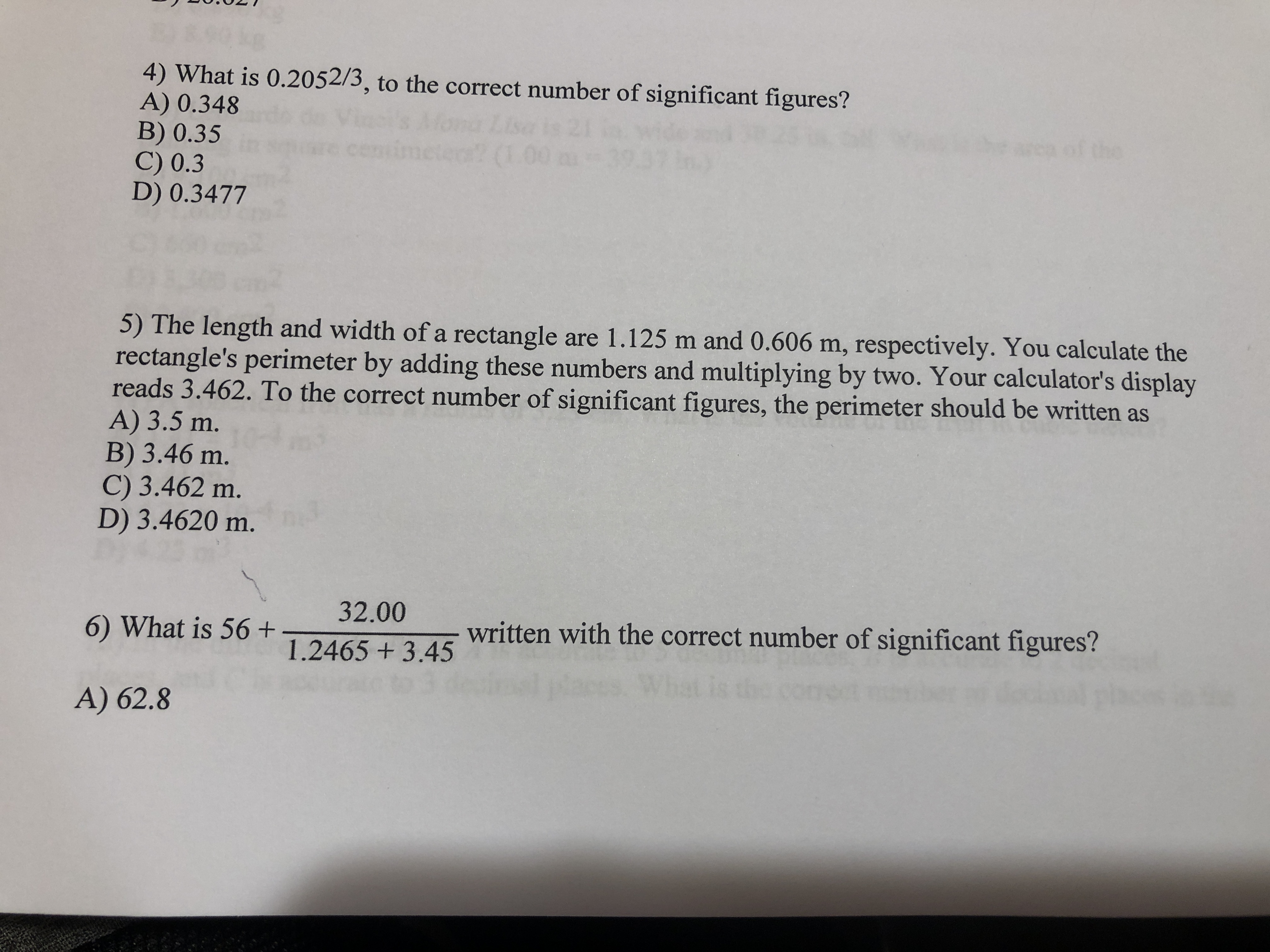# 4) What is 0.2052/3, to the correct number of significant figures?A) 0.348B) 0.35C) 0.3D) 0.3477of thecento? (1.005) The length and width of a rectangle are 1.125 m and 0.606 m, respectively. You calculate therectangle's perimeter by adding these numbers and multiplying by two. Your calculator's displayreads 3.462. To the correct number of significant figures, the perimeter should be written asA) 3.5 m.B) 3.46 m.C) 3.462 m.D) 3.4620 m.32.006) What is 56+written with the correct number of significant figures?1.2465 +3.45A) 62.8

Question
10 viewshelp_outlineImage Transcriptionclose4) What is 0.2052/3, to the correct number of significant figures? A) 0.348 B) 0.35 C) 0.3 D) 0.3477 of the cent o? (1.00 5) The length and width of a rectangle are 1.125 m and 0.606 m, respectively. You calculate the rectangle's perimeter by adding these numbers and multiplying by two. Your calculator's display reads 3.462. To the correct number of significant figures, the perimeter should be written as A) 3.5 m. B) 3.46 m. C) 3.462 m. D) 3.4620 m. 32.00 6) What is 56+ written with the correct number of significant figures? 1.2465 +3.45 A) 62.8 fullscreen
check_circle

Step 1

Find 0.2052/3.

Step 2

Rounding it to the correct sig...

### Want to see the full answer?

See Solution

#### Want to see this answer and more?

Solutions are written by subject experts who are available 24/7. Questions are typically answered within 1 hour.*

See Solution
*Response times may vary by subject and question.
Tagged in

### Units and Dimensions